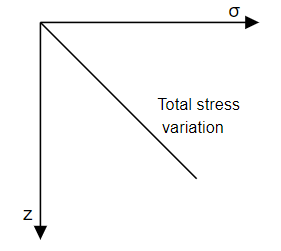top of page
Search
• APSEd

# Total Stress and Effective Stress on Soil with Concepts and Formulas

Updated: Jan 30, 2022

Total stress at a layer present at a certain depth below ground level is the total weight of the soil present above that layer per unit surface area of the soil mass. Effective stress is the part of the total stress that is resisted by soil particles by grain-to-grain interaction. The concept and methods of computing total and effective stress are discussed further.

## Total Stress Concept

As said earlier, total stress at a layer is the total weight of the soil present above that layer, including self-weight of water present in soil pores or present above the ground level, per unit surface area of the soil. Total stress also includes the stresses de to externally applied loads, if any.

The pattern of stresses due to self-weight could be complicated except for one common case where the stresses are simple. One common case is when the ground surface is horizontal and the nature of the soil does not vary significantly in the horizontal directions. This is the most frequent case in any sedimentary deposit. Also, in this case, there can be no shear stresses upon vertical and horizontal planes within the soil mass.

Therefore, for the above-said case, total stress at a layer present at a certain depth can be computed as the product of the weight of the soil and depth below ground level at which total stress is required. Thus, the total stress varies linearly with depth.Total stress distribution

### Total Stress Formula

Total stress (σv) at a layer present at a depth of 'z' from the ground surface is given by,

σ = γ * z,

provided unit weight (γ) of the soil is constant.

where,

σ - total vertical stress (kN/m^2),

γ - the unit weight of soil (kN),

z - depth under consideration (m).

## Pore Water Pressure Concept

As we all know, the soil is a three-phase system i.e., it contains solid, porewater and air voids. In the case of saturated soils, the pressure exerted by the pore water to its surrounding solid mass is called pore water pressure. Pore water pressure is also called Neutral stress.

Neutral stress can be defined as the stress carried (exerted) by the pore water and it is the same in all directions when there is static equilibrium.

### Pore Water Pressure Formula

Pore water pressure(u) at a depth z can be calculated as the product of the unit weight of water and depth as given below.

u = γw * z,

where,

γw - the unit weight of the water (9.81 kN/m^3),

z - depth at which pore water pressure is required.

Pore water pressure can become negative in the case of capillary water. In such a case, pore water pressure (u) is calculated as,

u = -γw * h,

where,

h - the height of the capillary rise above the ground level

To know more about capillary water and its effect on effective stress, refer to the video lecture here.

## Effective Stress Concept

The total stress (that was discussed earlier) at any point inside the soil is resisted by the soil grains and also by water present in the pores or void spaces (in the case of saturated soils). This portion of total stress that is resisted by soil grains is called effective stress. Effective stress is in macroscopic scale i.e., force/total area, and not in terms of contact stress at the grain-to-grain contacts as these will be very high.

In simple terms, imagine total stress as downward stress that is being resisted by effective stress by virtue of soil grains and pore water pressure by virtue of pore water, both acting in the upward direction.

### Effective Stress Formula

Effective stress cannot be directly calculated but it can be calculated as the difference between total stress(σ) and pore water pressure(u). It is represented using σ'.

Effective stress, σ' = Total stress (σ) - Pore water pressure(u)

## Example problem

Question: Determine total, neutral, and effective stress at a depth of 16m below the ground. The water table is 4m below the ground level. Take γbulk = 16.5 kN/m^3, and γsat = 19.4 kN/m^3.

Solution:

Take z1 = 4m, and z2 = 12m (i.e., 16 - 4)

Total stress at depth of 4m, σ = γbulk * z1

σ = 16.5 * 4 = 66 kN/m^2

Total stress at depth of 16m, σ = γbulk * z1 + γsat * z2

σ = 16.5 * 4 + 19.4 * 12 = 298.8 kN/m^2

Neutral stress at depth of 16m, u = γw * z2

u = 9.81*12 = 117.72 kN/m^2

Effective stress at a depth of 4m, σ' = σ - u

σ' = 66 - 0 = 66 kN/m^2

Effective stress at a depth of 16m, σ' = σ - u

σ' = 298.8 - 117.72 = 181.08 kN/m^2

Note: All units are in kN/m^2 in the below-given diagram.Total, neutral and effective distribution

## Practise problem

Do check out the below-given video lecture on tricky effective stress problems.

Want us to cover a topic of your interest? Fill out the form below and let us know :)